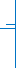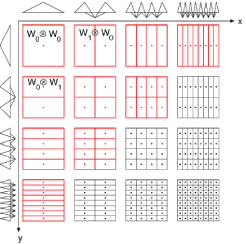// ZSS 2010 Zuerich Summer School 201023. - 27.08.2010## Sparse Tensor Discretizations of High-Dimensional Problems

### Zurich, 23. - 27.08.2010

Pictures GalleryPlenary Lectures: L. Grasedyck (MPI Leipzig, Germany) M. Griebel (University of Bonn, Germany) R. Hiptmair (ETH Zurich, Switzerland) M. Mohlenkamp (Ohio University, USA) Ch.Schwab (ETH Zurich, Switzerland) Themes: Low rank approximations in numerical linear algebra Adaptivity in tensor discretizations Hyperbolic cross and sparse grid approximation Regularity of high-dimensional PDE problems Complexity of sparse approximation methods Low rank discretizations of operator equations Applications: PDE sensitivity on high-dimensional parameter spaces Stochastic partial differential equations High-dimensional transport equations Electron structure calculations